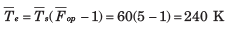Courses

# Test: Noise

## 20 Questions MCQ Test RRB JE for Electronics & Communication Engineering | Test: Noise

Description
This mock test of Test: Noise for Electronics and Communication Engineering (ECE) helps you for every Electronics and Communication Engineering (ECE) entrance exam. This contains 20 Multiple Choice Questions for Electronics and Communication Engineering (ECE) Test: Noise (mcq) to study with solutions a complete question bank. The solved questions answers in this Test: Noise quiz give you a good mix of easy questions and tough questions. Electronics and Communication Engineering (ECE) students definitely take this Test: Noise exercise for a better result in the exam. You can find other Test: Noise extra questions, long questions & short questions for Electronics and Communication Engineering (ECE) on EduRev as well by searching above.
QUESTION: 1

### An amplifier has a standard spot noise figure F0  = 6.31 (8.0 dB). The amplifier, that is used to amplify the output of an antenna have antenna temperature of Ta = 180 K Que: The effective input noise temperature of this amplifier is

Solution: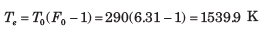QUESTION: 2

### An amplifier has a standard spot noise figure F0  = 6.31 (8.0 dB). The amplifier, that is used to amplify the output of an antenna have antenna temperature of Ta = 180 K Que: The operating spot noise figure is

Solution: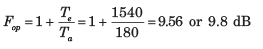QUESTION: 3

### An amplifier has three stages for which Te1 = 200 K (first stage), Te2  = 450 K, and Te3 = 1000K (last stage). If the available power gain of the second stage is 5, what gain must the first stage have to guarantee an effective input noise temperature of 250 K ?

Solution: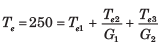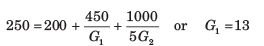QUESTION: 4

An amplifier has an operating spot noise figure of 10 dB when driven by a source of effective noise temperature 225 K.

Que: The standard spot noise figure of amplifier is

Solution: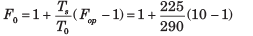= 7.98 or 9.0 dB

QUESTION: 5

An amplifier has an operating spot noise figure of 10 dB when driven by a source of effective noise temperature 225 K.

Que: If a matched attenuator with a loss of 3.2 dB isplaced between the source and the amplifier's input,what is the operating spot noise figure of the attenuator's amplifier cascade if the attenuator's physicaltemperature is 290 K ?

Solution: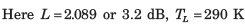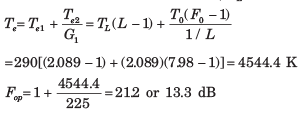QUESTION: 6

An amplifier has an operating spot noise figure of 10 dB when driven by a source of effective noise temperature 225 K.

Que: In previous question what is the standard spot noisefigure of the cascade ?

Solution: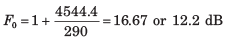QUESTION: 7

Omega Electronics sells a microwave receiver

(A) having an operating spot noise figure of 10 dB when driven by a source with effective noise temperature 130 K Digilink

(B) sells a receiver with a standard spot noise figure of 6 dB. Microtronics

(C) sells a receiver with standard spot noise figure of 8 dB when driven by a source with effective noise temperature 190 K. The best receiver to purchase is

Solution: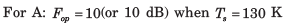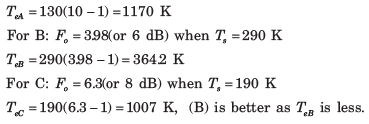QUESTION: 8

An amplifier has three stages for which Te1 = 150 K (first stage), Te3 = 350 K, and Te3 = 600 K (output stage). Available power gain of the first stage is 10 and overall input effective noise temperature is 190 K

Que: The available power gain of the second stage is

Solution: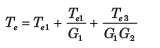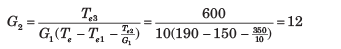QUESTION: 9

An amplifier has three stages for which Te1 = 150 K (first stage), Te3 = 350 K, and Te3 = 600 K (output stage). Available power gain of the first stage is 10 and overall input effective noise temperature is 190 K

Que: The cascade's standard spot noise figure is

Solution: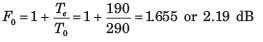QUESTION: 10

An amplifier has three stages for which Te1 = 150 K (first stage), Te3 = 350 K, and Te3 = 600 K (output stage). Available power gain of the first stage is 10 and overall input effective noise temperature is 190 K

Que: What is the cascade's operating spot noise figure when used with a source of noise temperature Ts = 50 K

Solution: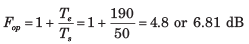QUESTION: 11

Three network are cascaded. Available power gains are G1 = 8, G2 = 6 and G3 = 20. Respective input effective spot noise temperature are Te1 = 40 K, Te2 = 100 K and Te3 =180 K.

Solution: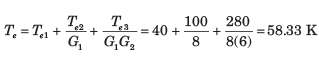QUESTION: 12

Three identical amplifier, each having a spot effective input noise temperature of 125 K and available power G are cascaded. The overall spot effective input noise temperature of the cascade is 155 K. The G is

Solution: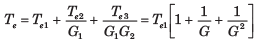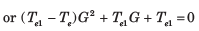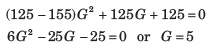QUESTION: 13

Three amplifier that may be connected in any order in a cascade are defined as follows: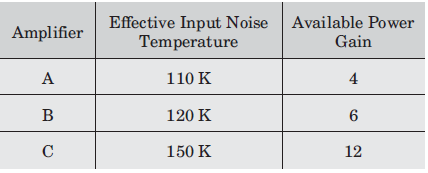The sequence of connection that will give the lowest overall effective input noise temperature for the cascade is

Solution:

Sequence Te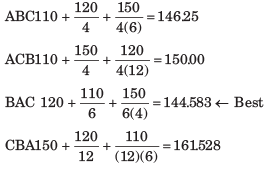QUESTION: 14

What is the maximum average effective input noise temperature that an amplifier can have if its average standard noise figure is to not exceed 1.7 ?

Solution: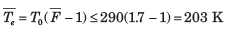QUESTION: 15

An amplifier has an average standard noise figure of 2.0 dB and an average operating noise figure of 6.5 dB when used with a source of average effective source temperature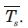Theis

Solution: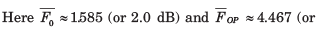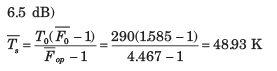QUESTION: 16

An antenna with average noise temperature 60 K connects to a receiver through various microwave elements that can be modeled as an impedance matched attenuator with an overall loss of 2.4 dB and a physical temperatures of 275 K. The overall system noise temperature of the receiver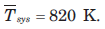Que: The average effective input noise temperature of the receiver is

Solution: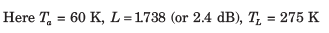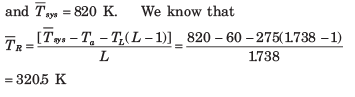QUESTION: 17

An antenna with average noise temperature 60 K connects to a receiver through various microwave elements that can be modeled as an impedance matched attenuator with an overall loss of 2.4 dB and a physical temperatures of 275 K. The overall system noise temperature of the receiverSolution: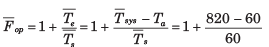= 13.67 or 11.4 dB

QUESTION: 18

If receiver has an available power gain of 110 dB and a noise band width of 10 MHz, the available output noise power of receiver is

Solution: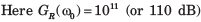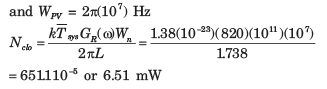QUESTION: 19

If antenna attenuator cascade is considered as a noise source, its average effective noise temperature is

Solution: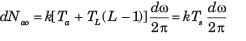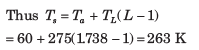QUESTION: 20

An amplifier when used with a source of average oise temperature 60 K, has an average operating noise figure of 5.
Que: The Te is

Solution: